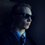# Logical Transformations as a Klein 4-Group

After recently studying statements and their logical transformations, I was happily surprised to discover a resemblance between these transformations and a special type of group known in group theory as a Klein 4-group.

First off, the logical transformations I was studying were the contrapositive, the converse, and the inverse. For example, if we take the statement "If x then y", we can transform it into:

1. The contrapositive: "If not y, then not x",
2. The converse: "If y, then x", and
3. The inverse: "If not x, then not y"

For convenience, I will abbreviate these $C_P$, $C$, and $I$, and the original statement $S$.

In exploring these transformations further, I realized that instead of going from the original statement to one of the transformations, I could apply a transformation on top of a transformation.

For example, the contrapositive of the converse is the contrapositive of "If y, then x", which is "If not x, then not y".

However, these double transformations all became other transformations! In the example above, for instance, the resulting statement is really just the inverse of the original statement. After trying out many of these double transformations, I realized that I was actually constructing a Cayley table.

Without delving to deeply into group theory, a Cayley table is a table used to show how a collection of items behave when combined in certain ways. In my case, I realized I could use a Cayley table to show how logic transformations behaved when applied more then once on a statement.

I came up with the following table:

 $~$ $S~$ $C_P$ $C~$ $I~$ $S~$ $S~$ $C_P$ $C~$ $I~$ $C_P$ $C_P$ $S~$ $I~$ $C~$ $C~$ $C~$ $I~$ $S~$ $C_P$ $I~$ $I~$ $C~$ $C_P$ $S~$

Which has been classified, more generally, by group theorists as the Klein 4-group. Technically, this represents a transformation in any row first being applied, and then a transformation in any column being applied second with their intersection being the resulting statement. However, as is the case with this and many other types of groups, the transformations are commutative, meaning the order they are applied in does not matter.

Maybe it is just me, but I thought it so fascinating that something as fundamental as logic could be described by something so abstract as group theory, and thus relating it to a host of other applications such as physics, chemistry, and Rubik's cubes!

I would love any feedback anyone has on this topic. I have not been able to find anything online relating logic to group theory, so any extra information or ideas would be much appreciated!Note by David Stiff
2 years, 7 months ago

This discussion board is a place to discuss our Daily Challenges and the math and science related to those challenges. Explanations are more than just a solution — they should explain the steps and thinking strategies that you used to obtain the solution. Comments should further the discussion of math and science.

When posting on Brilliant:

• Use the emojis to react to an explanation, whether you're congratulating a job well done , or just really confused .
• Ask specific questions about the challenge or the steps in somebody's explanation. Well-posed questions can add a lot to the discussion, but posting "I don't understand!" doesn't help anyone.
• Try to contribute something new to the discussion, whether it is an extension, generalization or other idea related to the challenge.

MarkdownAppears as
*italics* or _italics_ italics
**bold** or __bold__ bold
- bulleted- list
• bulleted
• list
1. numbered2. list
1. numbered
2. list
Note: you must add a full line of space before and after lists for them to show up correctly
paragraph 1paragraph 2

paragraph 1

paragraph 2

[example link](https://brilliant.org)example link
> This is a quote
This is a quote
    # I indented these lines
# 4 spaces, and now they show
# up as a code block.

print "hello world"
# I indented these lines
# 4 spaces, and now they show
# up as a code block.

print "hello world"
MathAppears as
Remember to wrap math in $$ ... $$ or $ ... $ to ensure proper formatting.
2 \times 3 $2 \times 3$
2^{34} $2^{34}$
a_{i-1} $a_{i-1}$
\frac{2}{3} $\frac{2}{3}$
\sqrt{2} $\sqrt{2}$
\sum_{i=1}^3 $\sum_{i=1}^3$
\sin \theta $\sin \theta$
\boxed{123} $\boxed{123}$

Sort by:

This is a result of the fact that inverse and converse are both C2 groups and combining both of them is equivalent to taking a direct sum on some level.

- 1 year, 3 months ago

Thanks Sam. I must admit that I am not very knowledgeable in the field of group theory. When I posted this, I merely discovered a resemblance to something called the Klein-4 group which I had read about briefly.

- 1 year, 3 months ago

I don't think this is a particularly surprising result, as every group of order 4 is either cyclic or isomorphic to the Vierergruppe... Obviously it's not cyclic (since each logical statement is idempotent); hence, the only thing to verify is closure and associativity of composition of "logical transformations" (whatever that means). There's also an application of the Vierergruppe to music composition too...

I think it would be beneficial for you to add this to the Klein 4-group Wikipedia page, as well as this page, but you may want to make sure that your writing is perfect.

- 2 years, 7 months ago